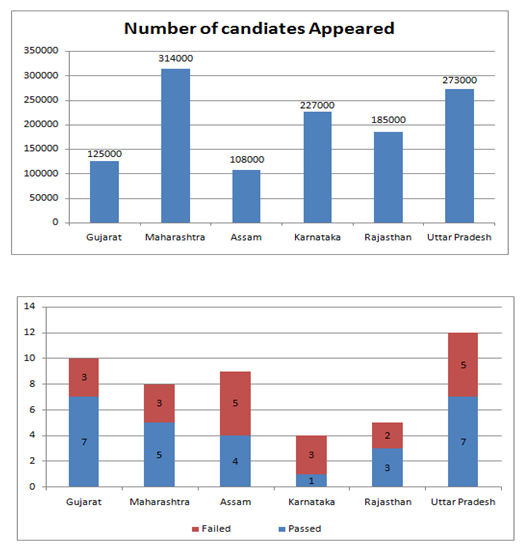# “20-20” Quantitative Aptitude | Crack SBI Clerk 2018 Day-6

Dear Friends, SBI Clerk 2018 Notification has been released we hope you all have started your preparation. Here we have started New Series of Practice Materials specially for SBI Clerk 2018. Aspirants those who are preparing for the exams can use this “20-20” Quantitative Aptitude Questions.

[WpProQuiz 1146]

Click “Start Quiz” to attend these Questions and view Explanation

### Click Here for SBI Clerk Prelims and Mains 2018 – Full Length Mock Test

Directions (Q. 1-5): What approximate value should come in place of question mark (?) in the following questions (?) (you are not expected to calculate the exact value).

1). 180% of 25501 + 50% of 28999 – 7634.97 = ?

1. 56870
2. 52770
3. 57720
4. 53875
5. 57270

2). 174.995 × 14.995 ÷ 25 + ? + 86.93 × 3.004 = 495

1. 220
2. 180
3. 105
4. 340
5. 130

3). 140% of 56 + 56% of 140.003 – √2026 – ? = 40

1. 40
2. 220
3. 70
4. 330
5. 180

4). 5687.285 + 4872.35 ÷ 12 × 6.989 = 5 × (3699.98 – ?)

1. 1740
2. 2096
3. 1910
4. 1860
5. 2000

5). 1325√17 + 20% of ? – 83.99 of (3/4) = 5500

1. 520
2. 1280
3. 1320
4. 680
5. None of these

Directions (Q. 6-10): what will come in place of question mark (?) in the given number series?

6). 4,  11,  24,  44,  72,  ?

1. 121
2. 113
3. 109
4. 118
5. 105

7). 13,  21,  37,  69,  ?   261

1. 133
2. 125
3. 141
4. 139
5. None of these

8). 17,  25,  15,  27,  13,  ?

1. 29
2. 31
3. 27
4. 35
5. 33

9). 11,  12,  20,  29,  93,  ?

1. 104
2. 116
3. 108
4. 120
5. 118

10). 11,  12,  26,  81,  328,  ?

1. 1720
2. 1645
3. 1540
4. 1625
5. 1575

Directions (Q. 11-15): Study the graphs carefully and answer the questions that follow:

The bar graph shows the number of candidates appeared in IBPS RRB Office Assistant examination from six different states and another bar graph shows the ratio of candidates passed and failed in the states1. What is the respective ratio of the number of candidates failed in the Exam from Karnataka and Rajasthan together to those failed in the exam from Gujarat and Maharashtra together?
1. 927 : 623
2. 621 : 977
3. 977 : 621
4. 947 : 459
5. 927 : 611
1. The number of candidates passed from Rajasthan and Assam together is what percent of the total number of failed candidates from Karnataka?
1. 95.78%
2. 91.28%
3. 95.49%
4. 92.01%
5. 93.39%
1. The number of candidates appearing for the exam from Assam and Gujarat together is what percent of the total number of candidates appearing for the exam from Maharashtra and Uttar Pradesh together? (rounded off to the nearest integer)
1. 45
2. 40
3. 35
4. 33
5. 30
1. Number of candidates passing in the exam from Uttar Pradesh is what percent of the total number of candidates appearing from all the States together? (rounded off to two digits after the decimal)
1. 12.93
2. 11.46
3. 13.84
4. 15.27
5. 14.52
1. What is the difference between the state has the highest number of passing students in the exam to the state has the lowest number of students failed in the exam?
1. 132250
2. 168350
3. 164450
4. 158750
5. 148950

16).The ratio of present ages of son and daughter of a lady is in the ratio of 7:8 and the age of lady is sum total of ages of her daughter and son. If the ratio of ages of son after 3 years and the age of daughter 6 years ago is 4 : 3 then what will be the ratio of the present age of lady and the age of her son after four years?

1. 4:5
2. 5:3
3. 6:5
4. 9:5
5. None of these

17).In a two digit number the digit at unit’s place is less than twice the digit at ten’s place by 1. And the difference between the number obtained by interchanging its digits and the original number is 50% of the number obtained by adding 1 in original number. What is the original number?

1. 46
2. 57
3. 35
4. 68
5. None of these

18).An alloy contains mixture of two metals A and B in the ratio of 3:5, and the second alloy contains, mixture of same metals in the ratio of 2.33 : 3. In what ratio should be both alloy mixed to make a new alloy containing 42 6/7 % metal A?

1. 1 : 2
2. 3 : 2
3. 2 : 3
4. 1 : 6
5. None of these

19).The profit earned by a company is to be distributed among the managers and officers in the ratio of 7 : 5. If the number of managers and officers is in the ratio of 7 : 12 the amount received by each officers is 30,000 what was the total profit earned if the number of managers was 28?

1. 35 lakh
2. 17.5 lakh
3. 34.56 lakh
4. 19 lakh
5. None of these

20). A shopkeeper offers two successive discounts of 10% and 15% on an article and is still able to earn 53% profit. If he offers only 20% discount what will be his profit percentage?

1. 70%
2. 60%
3. 65%
4. 80%
5. None of these